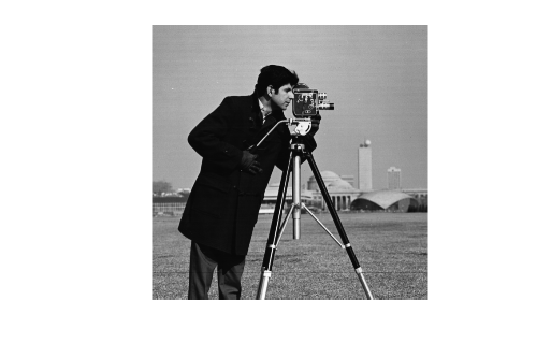# getImage

Read images stored in MATLAB interface object for OpenCV class

Since R2021b

## Syntax

``outputImg = getImage(ocvInput)``

## Description

example

````outputImg = getImage(ocvInput)` reads the image stored in a MATLAB® interface object for an OpenCV class and writes it to the specified output variable `outputImg` in the MATLAB workspace.```

## Examples

collapse all

Add the MATLAB interface to OpenCV package names to the import list.

```import clib.opencv.* import vision.opencv.util.*```

Read an image into the MATLAB workspace.

`img = imread("cameraman.tif");`

Create a MATLAB interface object for the OpenCV `InputOutput` array class.

`[ocvMat,ocvArray] = createMat(img);`

Read the image stored in the `InputOutput` interface object.

`output = getImage(ocvArray);`

Display the output image.

```figure imshow(output)```## Input Arguments

collapse all

MATLAB interface object for an OpenCV class, specified as one of these values:

• `MatND` interface object — Created by using the `createMat` function and represents the OpenCV `cv::Mat` class.

• `UMat` interface object — Created by using the `createUMat` function and represents the OpenCV `cv::UMat` class.

• `InputArray` interface object — Created by using the `createMat` or `createUMat` function and represents the OpenCV `cv::_InputArray` class. The `InputArray` interface object passes array inputs to the OpenCV functions.

• `InputOutputArray` interface object — Created by using the `createMat` or `createUMat` function and represents the OpenCV `cv::_InputOutputArray` class. The `InputOutputArray` interface object passes array inputs to the OpenCV functions and writes the array outputs returned by the OpenCV functions.

• `OutputArray` interface object — Created by using the `createMat` or `createUMat` function and represents the OpenCV `cv::_OutputArray` class. The `OutputArray` interface object contains the array outputs returned by the OpenCV functions.

## Output Arguments

collapse all

Output image read from a MATLAB interface object, returned as an M-by-N matrix for intensity images and M-by-N-by-3 array for color images.

## Version History

Introduced in R2021b